# Contour Plots using Plotly in Python

• Last Updated : 12 Feb, 2021

A Plotly is a Python library that is used to design graphs, especially interactive graphs. It can plot various graphs and charts like histogram, barplot, boxplot, spreadplot, and many more. It is mainly used in data analysis as well as financial analysis. plotly is an interactive visualization library.

## Contour Plots in Plotly

A contour plot has a function of two variables of curves along which the function has constant values so that these curves join the points with equal values. In contour plot, a 2d contour plot presents contour lines of a 2D numerical array z, i.e. interpolated lines of iso values of z.

Syntax: plotly.graph_objects.Contour(arg=None,colorbar=None, hoverinfo=None, x=None,y=None,**kwargs)

Parameters:

arg: dict of properties compatible with this constructor or an instance of plotly.graph_objects.Contour

colorbar:  plotly.graph_objects.contour.ColorBar instance or dict with compatible properties

x: Sets the x coordinates.

y: Sets the y coordinates.

z: Sets the z coordinates.

hoverinfo: Determines which trace information appear on hover. If none or skip are set, no information is displayed upon hovering. But, if none is set, click and hover events are still fired.

Example 1: Creating a simple contour plot

## Python3

 `import` `plotly.graph_objects as go` `import` `numpy as np`   `data ``=` `[[``1``,``2``,``3``,``4``,``5``],` `     ``[``3``,``4``,``5``,``6``,``7``],` `     ``[``7``,``8``,``9``,``6``,``4``],` `     ``[``3``,``7``,``2``,``4``,``2``]]`   `fig ``=` `go.Figure(data ``=` `     ``go.Contour(z ``=` `data))`   `fig.show()`

Output: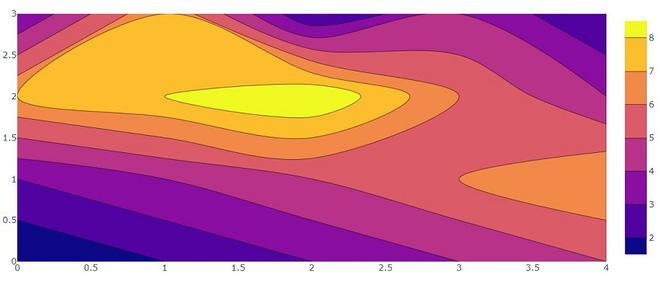Example 2: Creating contour plot with X and Y coordinates.

## Python3

 `import` `plotly.graph_objects as go`   `feature_x ``=` `np.arange(``0``, ``50``, ``2``) ` `feature_y ``=` `np.arange(``0``, ``50``, ``3``) `   `# Creating 2-D grid of features ` `[X, Y] ``=` `np.meshgrid(feature_x, feature_y) `   `Z ``=` `np.cos(X ``/` `2``) ``+` `np.sin(Y ``/` `4``) `   `fig ``=` `go.Figure(data ``=` `     ``go.Contour(x ``=` `feature_x, y ``=` `feature_y, z ``=` `Z))`   `fig.show()`

Output: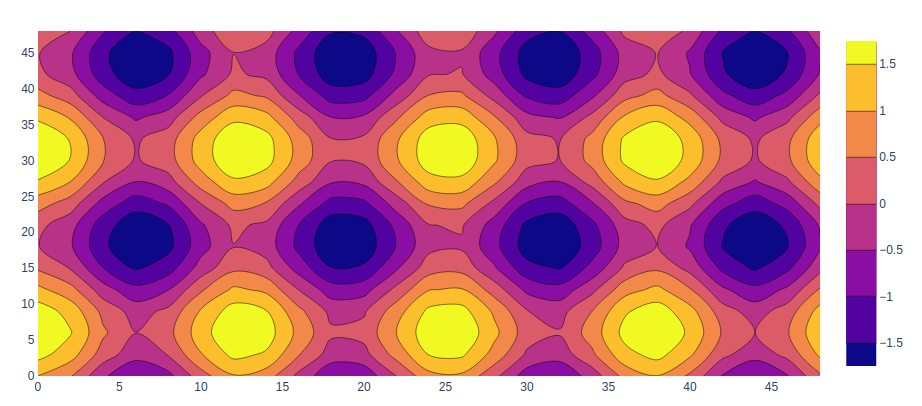In plotly, the colorscale for the contour plot is used to add different colors and can be set using the colorscale parameter.

Example:

## Python3

 `import` `plotly.graph_objects as go`   `feature_x ``=` `np.arange(``0``, ``50``, ``2``) ` `feature_y ``=` `np.arange(``0``, ``50``, ``3``) `   `# Creating 2-D grid of features ` `[X, Y] ``=` `np.meshgrid(feature_x, feature_y) `   `Z ``=` `np.cos(X ``/` `2``) ``+` `np.sin(Y ``/` `4``) `   `fig ``=` `go.Figure(data ``=` `     ``go.Contour(x ``=` `feature_x, y ``=` `feature_y, z ``=` `Z,` `               ``colorscale``=``'rainbow'` `               ``))`   `fig.show()`

Output: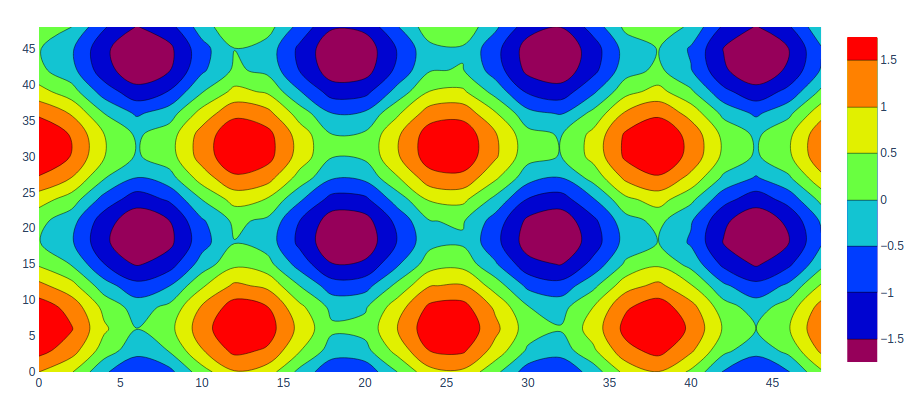### Custom Contour Plot Colorscale

Here we can customize our contour plot using colorscale. A list of colors that will be spaced evenly to create the colorscale. Many predefined colorscale lists are included in the sequential, diverging.

The following color set is available on colorscale.

‘aggrnyl’, ‘agsunset’, ‘algae’, ‘amp’, ‘armyrose’, ‘balance’, ‘blackbody’, ‘bluered’, ‘blues’, ‘blugrn’, ‘bluyl’, ‘brbg’,’brwnyl’, ‘bugn’, ‘bupu’, ‘burg’, ‘burgyl’, ‘cividis’, ‘curl’, ‘darkmint’, ‘deep’, ‘delta’, ‘dense’, ‘earth’, ‘edge’, ‘electric’, ’emrld’, ‘fall’, ‘geyser’, ‘gnbu’, ‘gray’, ‘greens’, ‘greys’,’haline’, ‘hot’, ‘hsv’, ‘ice’, ‘icefire’, ‘inferno’, ‘jet’, ‘magenta’, ‘magma’, ‘matter’, ‘mint’, ‘mrybm’, ‘mygbm’, ‘oranges’, ‘orrd’, ‘oryel’, ‘oxy’, ‘peach’, ‘phase’, ‘picnic’, ‘pinkyl’, ‘piyg’, ‘plasma’, ‘plotly3’, ‘portland’, ‘prgn’, ‘pubu’, ‘pubugn’,’puor’, ‘purd’, ‘purp’, ‘purples’, ‘purpor’, ‘rainbow’, ‘rdbu’, ‘rdgy’, ‘rdpu’, ‘rdylbu’, ‘rdylgn’, ‘redor’, ‘reds’, ‘solar’, ‘spectral’, ‘speed’, ‘sunset’, ‘sunsetdark’, ‘teal’, ‘tealgrn’, ‘tealrose’, ‘tempo’, ‘temps’, ‘thermal’, ‘tropic’, ‘turbid’, ‘turbo’, ‘twilight’, ‘viridis’, ‘ylgn’, ‘ylgnbu’, ‘ylorbr’, ‘ylorrd’].

Example:

## Python3

 `import` `plotly.graph_objects as go`   `feature_x ``=` `np.arange(``0``, ``50``, ``2``) ` `feature_y ``=` `np.arange(``0``, ``50``, ``3``) `   `colorscale ``=` `colorscale ``=` `[[``0``, ``'green'``], [``0.5``, ``'red'``],` `                           ``[``1.0``, ``'rgb(0, 0, 255)'``]]`   `# Creating 2-D grid of features ` `[X, Y] ``=` `np.meshgrid(feature_x, feature_y) `   `Z ``=` `np.cos(X ``/` `2``) ``+` `np.sin(Y ``/` `4``) `   `fig ``=` `go.Figure(data ``=` `     ``go.Contour(x ``=` `feature_x, y ``=` `feature_y, z ``=` `Z,` `               ``colorscale ``=` `colorscale))`   `fig.show()`

Output: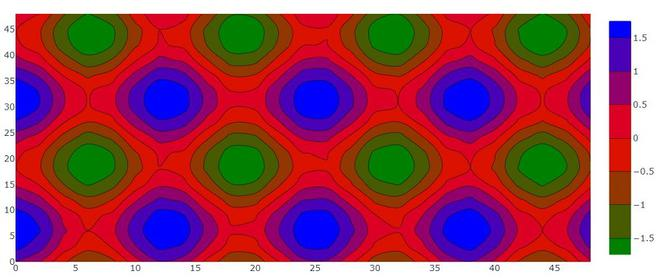### Customizing Size and Range

We can customize size and range of our contour plot using contours attributes. contours accept dict object that contains the range and size of the coordinates.

## Python3

 `import` `plotly.graph_objects as go`   `feature_x ``=` `np.arange(``0``, ``50``, ``2``) ` `feature_y ``=` `np.arange(``0``, ``50``, ``3``) `   `# Creating 2-D grid of features ` `[X, Y] ``=` `np.meshgrid(feature_x, feature_y) `   `Z ``=` `np.cos(X ``/` `2``) ``+` `np.sin(Y ``/` `4``) `   `fig ``=` `go.Figure(data ``=` `     ``go.Contour(x ``=` `feature_x, ` `                ``y ``=` `feature_y, z ``=` `Z,` `               `  `            ``contours``=``dict``(` `                ``start``=``1``,` `                ``end``=``5``,` `                ``size``=``.``2``)` `               ``)` `               ``)`   `fig.show()`

Output: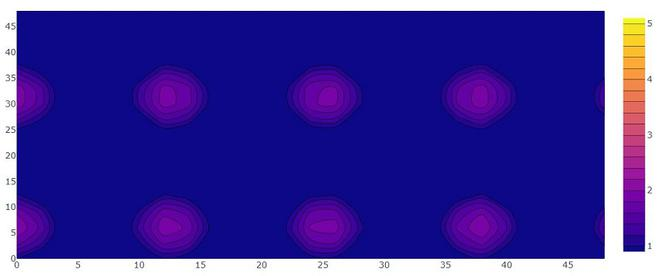### Adding Labels on Contour Line

Labels can be added using the contours parameter that has a property called showlabels. It determines whether to label the contour lines with their values.

Example:

## Python3

 `import` `plotly.graph_objects as go`   `feature_x ``=` `np.arange(``0``, ``50``, ``2``)` `feature_y ``=` `np.arange(``0``, ``50``, ``3``)`   `# Creating 2-D grid of features` `[X, Y] ``=` `np.meshgrid(feature_x, feature_y)`   `Z ``=` `np.cos(X ``/` `2``) ``+` `np.sin(Y ``/` `4``)`   `fig ``=` `go.Figure(data``=``go.Contour(` `    ``x``=``feature_x, y``=``feature_y, z``=``Z,` `    ``contours``=``dict``(` `        ``coloring``=``'lines'``,` `        ``showlabels``=``True``,)` `))`   `fig.show()`

Output: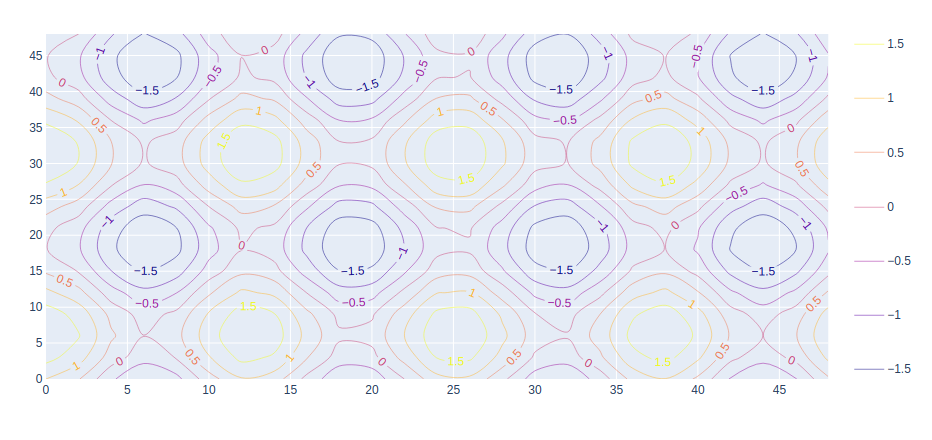My Personal Notes arrow_drop_up
Recommended Articles
Page :